We think you are located in Nigeria. Is this correct?

# Test yourself now

High marks in maths are the key to your success and future plans. Test yourself and learn more on Siyavula Practice.

# Chapter 14: Constructions

A construction in Mathematics is an accurate drawing of angles and lines. You are only allowed to use a straightedge and a pair of compasses with a pencil in it when you do constructions.

1. A straightedge

A straightedge is an instrument made of plastic, metal or wood that has a straight edge. You use it to draw straight lines. It is a ruler without any markings on it, because when you do constructions you are not allowed to take any measurements.Most of the time you will use your ruler when you do constructions and have to draw straight lines. The important thing to remember is that although there are markings on the ruler, you should not use them at all. You may only use the ruler to draw a straight line.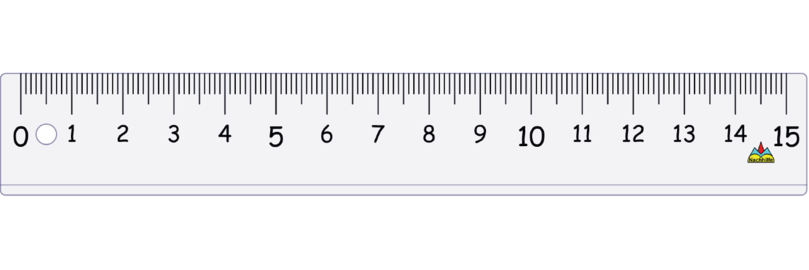2. A pair of compasses is an instrument to draw circles. It can also be called "a compass ".

A compass has two legs:

• One leg has a sharp point so that you can put it firmly into position on a piece of paper or page in a notebook. When you draw a circle the sharp point will stay on the dot where you positioned it. The sharp point will not move.
• The other leg has space to insert a pencil. When you draw a circle, you move this leg around, and the pencil will draw the circle on the page.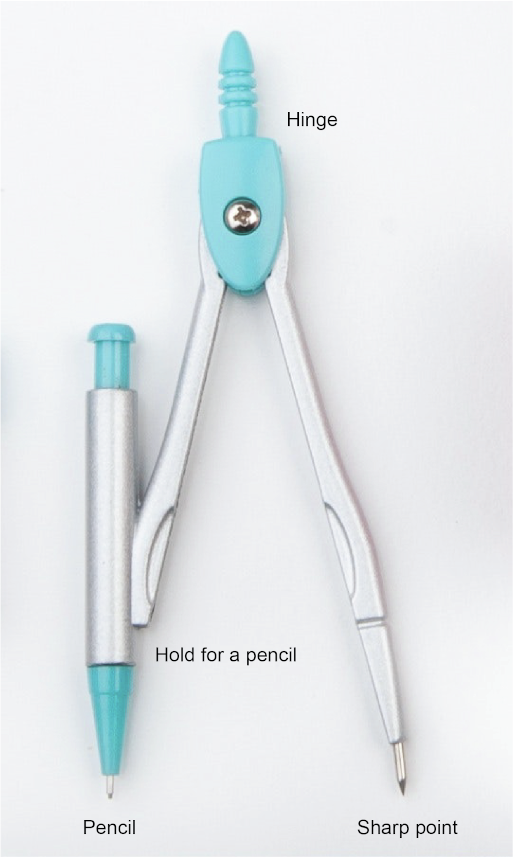The two legs of the compass are joined at the hinge. Make sure that the metal pin at the hinge is fitted tightly so that the two legs of the compass will not open too easily. Also make sure that your pencil is sharpened. A blunt pencil will lead to diagrams that are not accurate.

Do not confuse the compass that we use in mathematical constructions with the compass that we use to find direction.All the constructions in this chapter are based on the properties of a circle.

In the diagram below, $BC$ is the diameter of the circle. $A$ is the centre of the circle. This means that $AB$ is a radius of the circle and $AC$ is also a radius of the circle. So, $AB$ is equal to $AC$.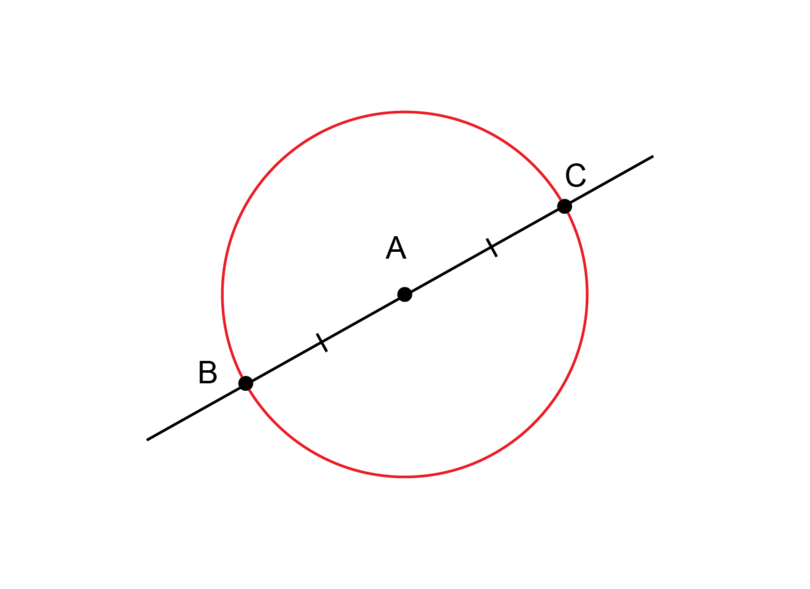When we do constructions we often do not draw a complete circle.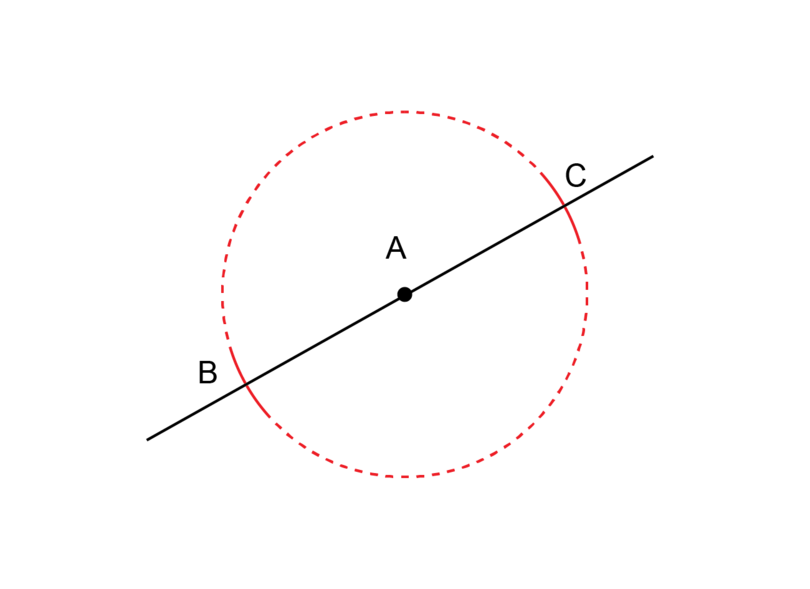The solid lines drawn at $B$ and $C$ are called arcs. An arc is part of the circumference of a circle.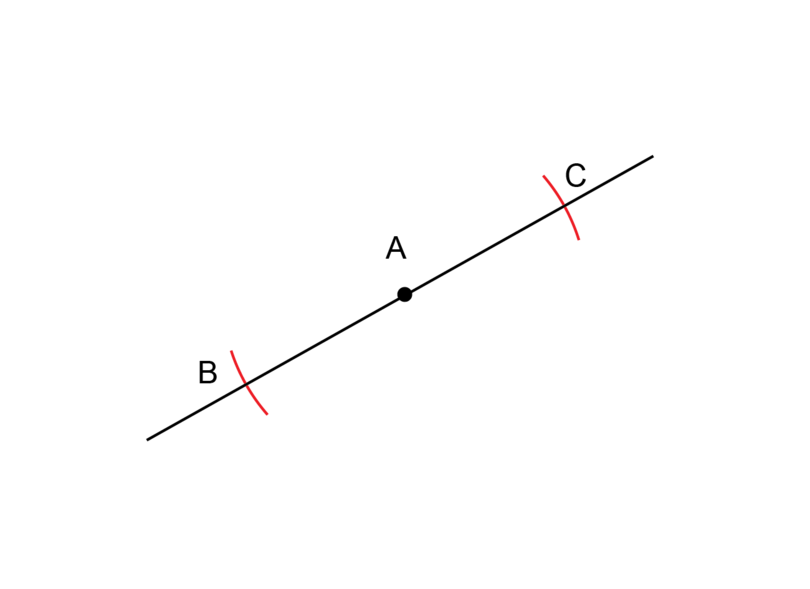We will draw arcs in all the constructions that we are going to do. It is important to remember that if we draw two arcs while our compass stays in the same position, we are drawing two radiuses with equal lengths. Even if we do not draw a complete circle, and we only draw an arc at $B$ and at $C$, $AB$ is still a radius of the circle and $AC$ is still a radius of the circle. So, $AB$ is equal to $AC$.

arc An arc is a part of the circumference of a circle.

## 14.1 Perpendicular lines

Lines that are perpendicular intersect each other at right angles (at $90^{\circ}$). When we are asked to construct a perpendicular line, we will be given one of the lines, as well as a point. We then have to construct a line through the given point so that the new line is perpendicular to the given line are perpendicular.

intersect When two lines intersect, they cross each other.

perpendicular line A perpendicular line cuts another line at a right angle.

The point that is given can be on the line, or it can be a small distance away from the line.In the first diagram, point $P$ is on line $AB$. In the second diagram, point $P$ is some distance away from line $AB$.

### Worked example 14.1: Constructing a perpendicular line from a point on the line

You are given line $AB$ with a point $P$ on the line.Construct line $PQ$ so that $PQ$ is perpendicular to $AB$.

1. Step 1: Mark equal distances to both sides of point $P$ on line $AB$.

Put the compass on point $P$ (where the blue dot is shown).

Draw an arc to the left of point $P$. Then, while keeping the compass open by exactly the same amount, draw an arc to the right of point $P$. These arcs should intersect (cross) line $AB$.2. Step 2: Draw an arc from the left.

Put the compass point on the arc at the left (where the orange dot is shown). Draw an arc so that it is above point $P$.3. Step 3: Draw an arc from the right.

Keep the compass exactly the same width open as for Step 2. Put the compass point on the arc at the right (where the purple dot is shown). Draw an arc so that it intersects the arc from Step 2.4. Step 4: Draw the perpendicular line.

Point $Q$ is where the two arcs from Step 2 and Step 3 are intersecting each other. Use a ruler and draw a line from point $P$ and through point $Q$.5. Step 5: Check your construction.

Use a protractor to measure $Q\hat{P}A$ and $Q\hat{P}B$.
If each of them is equal to $90^{\circ}$, you have made an accurate construction.

When copying the lines in order to do constructions, the lines only need to be more or less the same length as given in the questions, and in more or less the same direction. It is a good idea to rotate your workbook if you have to do a construction involving a line that is not horizontal on the page in front of you. Never erase the construction lines after you have completed a construction.

### Exercise 14.1: Construct a perpendicular line from a point on the line

1. Draw line $CD$ as shown below. $P$ is a point on the line.Construct $PQ$ perpendicular to line $CD$. Remember to check your work.

Follow the Steps as shown in Worked example 14.1.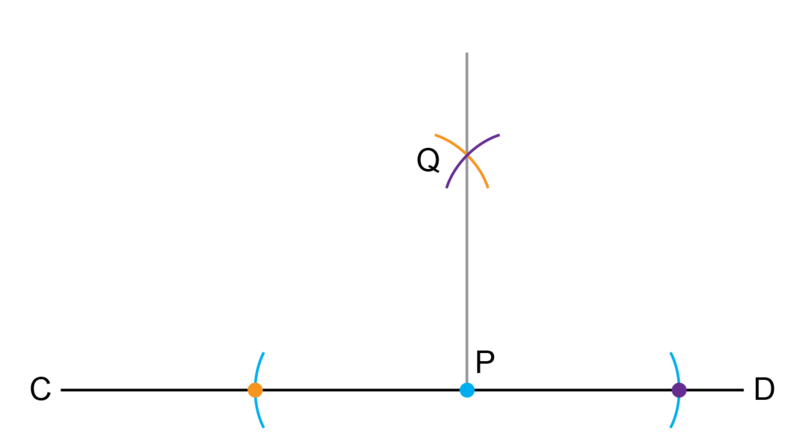If you have worked accurately, $Q \hat {P} C$ and $Q \hat{P}D$ will both be equal to $90^{\circ}$.

2. Draw line $EF$ as shown below. $P$ is a point on the line.Construct $PQ$ perpendicular to line $EF$. Remember to check your work.

Follow the Steps as shown in Worked example 14.1.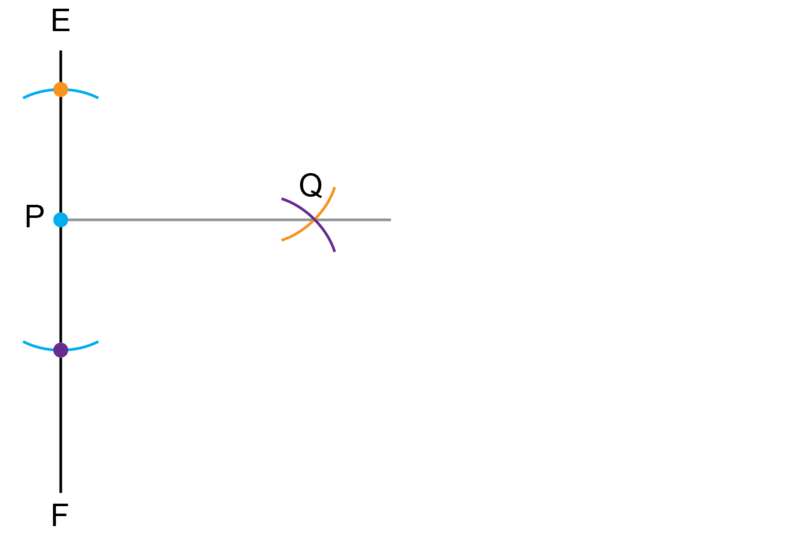If you have worked accurately, $Q\hat{P}E$ and $Q\hat{P}F$ will both be equal to $90^{\circ}$.

3. Draw line $MN$ as shown below. $P$ is a point on the line.Construct $PQ$ perpendicular to line $MN$. Remember to check your work.

Follow the Steps as shown in Worked example 14.1.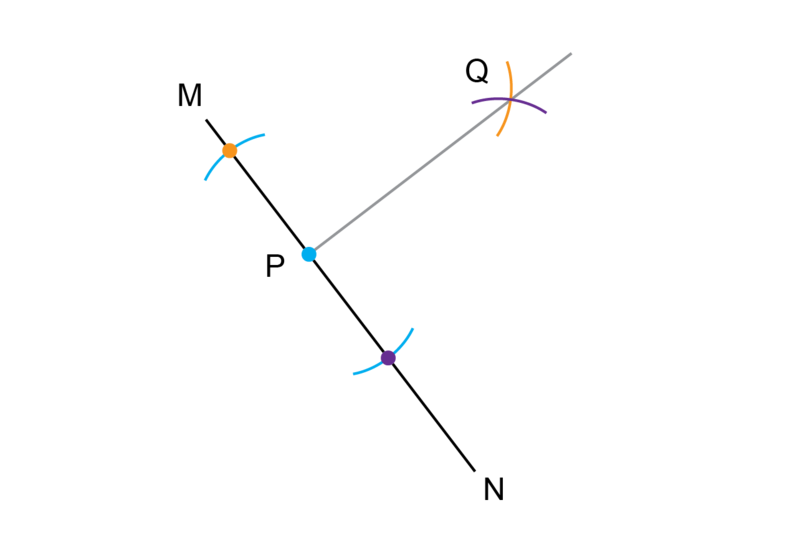If you have worked accurately, $Q\hat{P}M$ and $Q\hat{P}N$ will both be equal to $90^{\circ}$.

4. Draw line $ST$ as shown below. $P$ is a point on the line.Construct $PQ$ perpendicular to line $ST$. Remember to check your work.

Follow the Steps as shown in Worked example 14.1.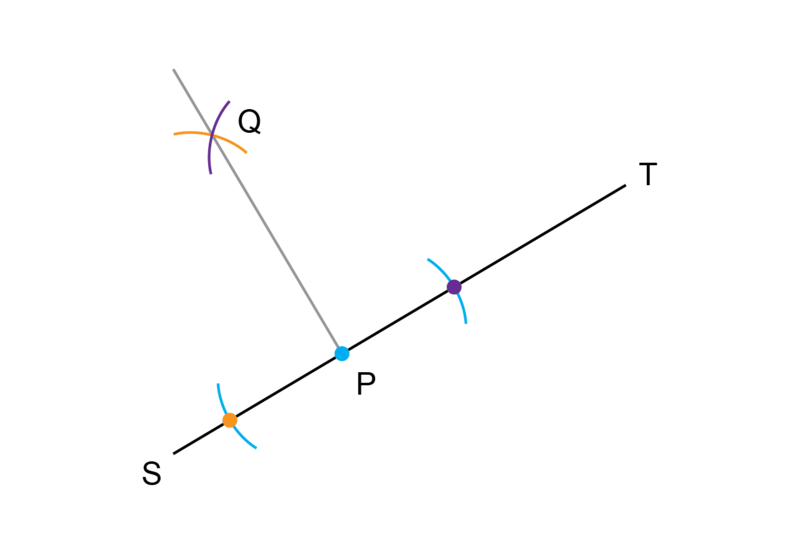If you have worked accurately, $Q\hat{P}S$ and $Q\hat{P}T$ will both be equal to $90^{\circ}$.

### Worked example 14.2: Constructing a perpendicular line from a point away from the line

You are given line $AB$ with a point $P$ away from the line.Construct line $PQ$ so that $PQ$ is perpendicular to $AB$.

1. Step 1: Mark equal distances from point $P$ on line $AB$.

Put the point of the compass on point $P$ (where the blue dot is shown).

Draw an arc to the left of point $P$ on the line. While keeping the compass exactly the same width open, draw an arc on the line to the right of point $P$. These arcs should intersect line $AB$.2. Step 2: Work from the left and draw an arc below line $AB$.

Put the compass point on the left arc of Step 1 (where the orange dot is shown). Draw an arc so that it is underneath point $P$.3. Step 3: Work from the right and draw a second arc below line $AB$.

Keep the compass the same width open as for Step 2. Put the compass point on the arc to the right arc of Step 1 (where the purple dot is shown). Draw an arc so that it crosses the arc from Step 2.4. Step 4: Draw the perpendicular line.

Point $Q$ is where the two arcs from Step 2 and Step 3 cross each other. Use a ruler and draw a line from point $P$ and through point $Q$.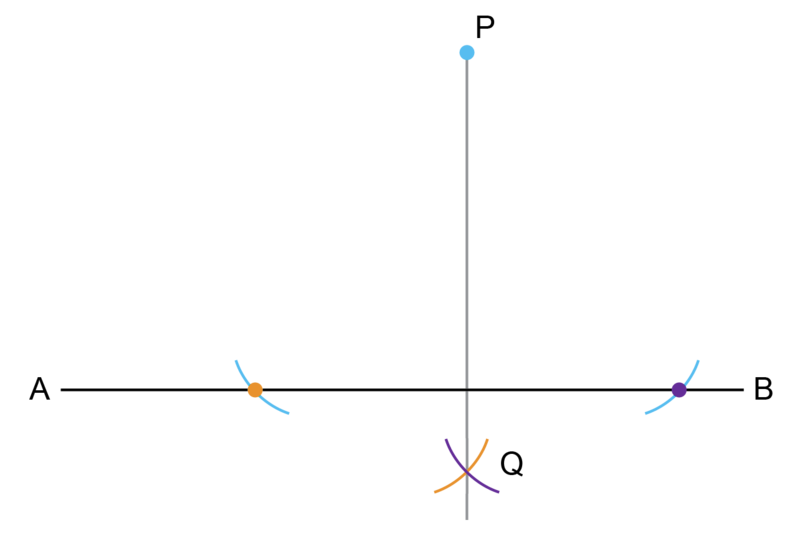5. Step 5: Check your construction.

Use a protractor and measure the angles where line $AB$ and line $PQ$ intersect. If each angle is equal to $90^{\circ}$, you have made an accurate construction.

Remember to rotate your workbook if you need to, and not to erase your construction lines. Also, always check your work.

### Exercise 14.2: Construct a perpendicular line from a point away from the line

1. Draw line $CD$ as shown below. Point $P$ is a point away from the line.Construct $PQ$ perpendicular to line $CD$.

Follow the Steps as shown in Worked example 14.2.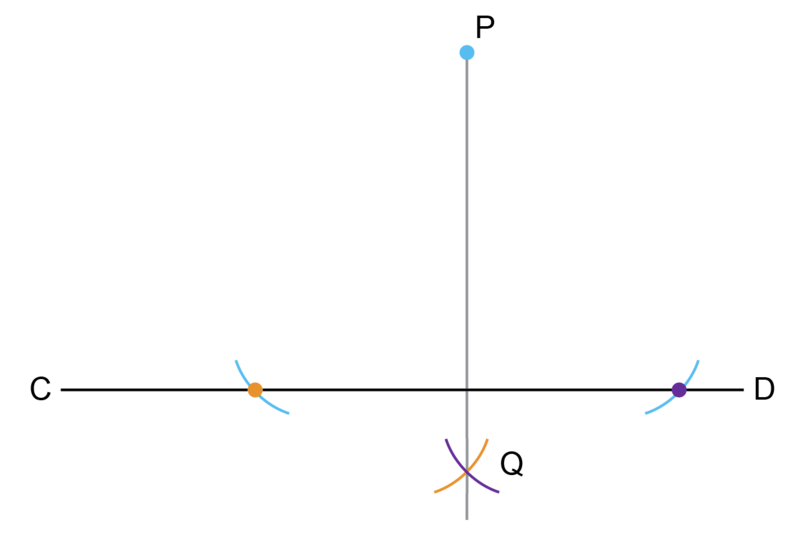If you have worked accurately, the two lines will intersect each other at an angle of $90^{\circ}$.

2. Draw line $EF$ as shown below. Point $P$ is a point away from the line.Construct $PQ$ perpendicular to line $EF$.

Follow the Steps as shown in Worked example 14.2.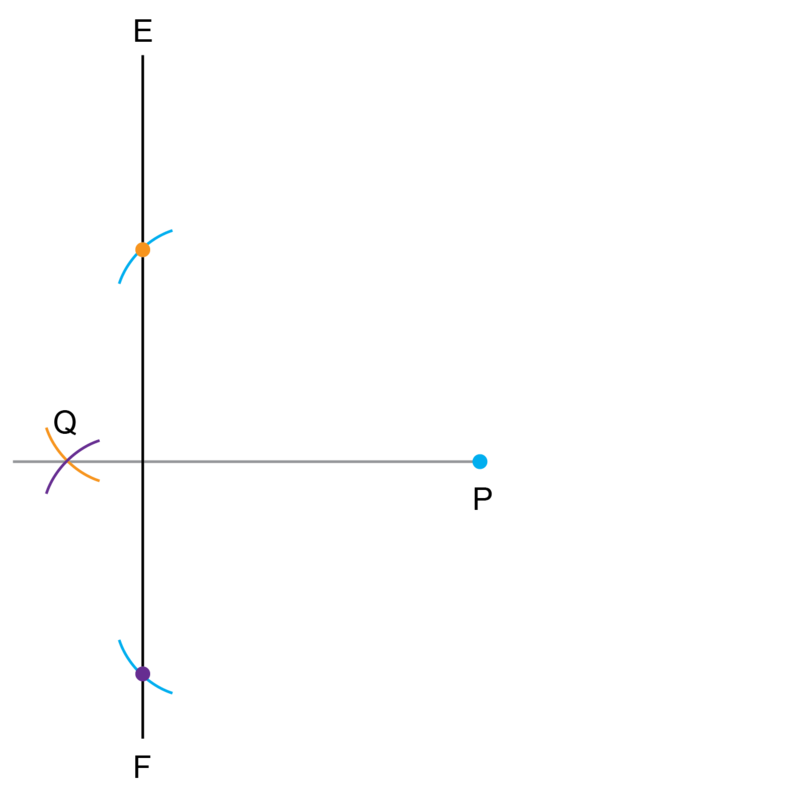If you have worked accurately, the two lines will intersect each other at an angle of $90^{\circ}$.

3. Draw line $MN$ as shown below. Point $P$ is a point away from the line.Construct $PQ$ perpendicular to line $MN$.

Follow the Steps as shown in Worked example 14.2.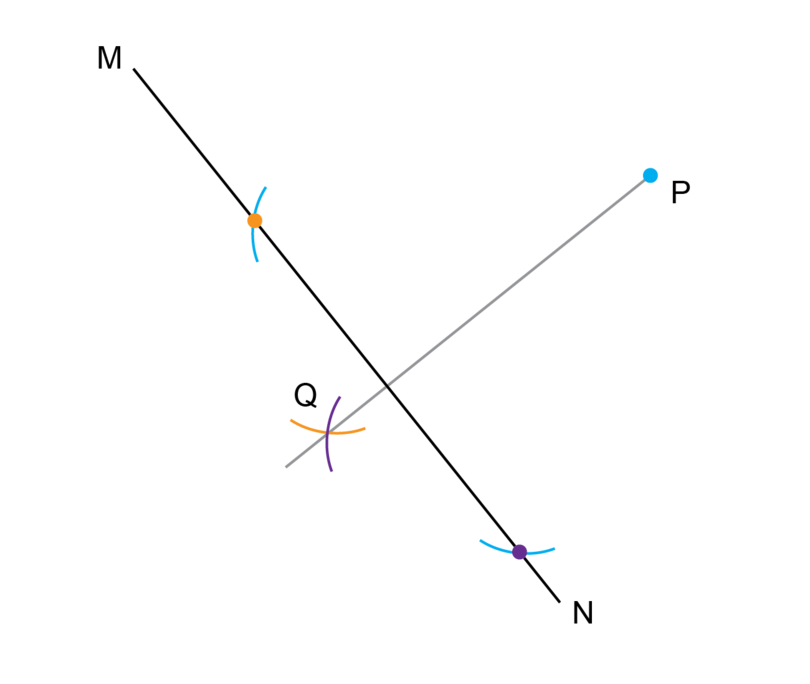If you have worked accurately, the two lines will intersect each other at an angle of $90^{\circ}$.

4. Draw line $ST$ as shown below. Point $P$ is a point away from the line.Construct $PQ$ perpendicular to line $ST$.

Follow the Steps as shown in Worked example 14.2.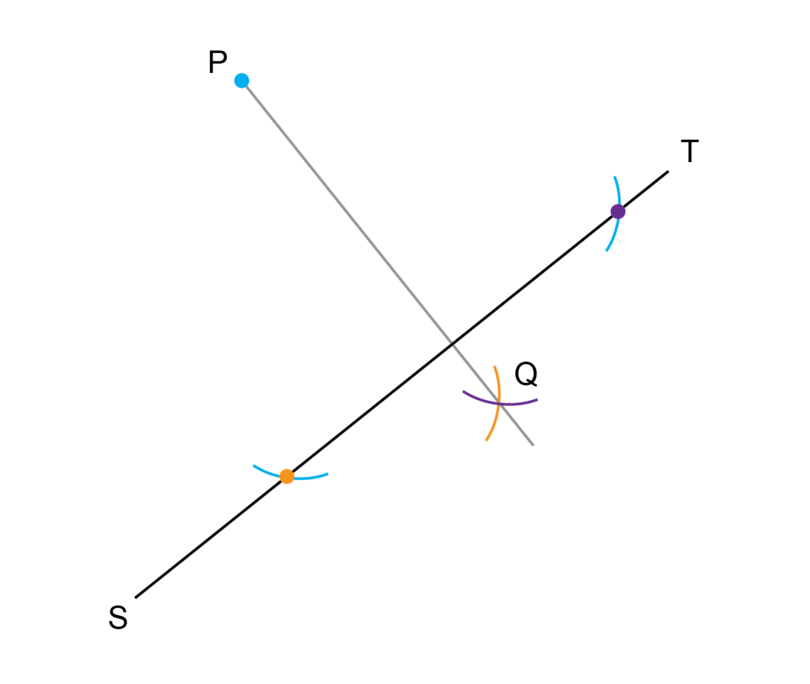If you have worked accurately, the two lines will intersect each other at an angle of $90^{\circ}$.

## 14.2 Parallel lines

Parallel lines are lines that are always the same distance apart from each other. They never meet or cross each other. When we are asked to construct parallel lines, we will be given a line and a point. We then have to construct a line through the given point, and then another line that is parallel to the first line.

parallel lines Parallel lines are lines that always remain the same distance apart, and never meet or cross each other.

You will learn more about parallel lines in Chapter 15, but it will be easier to construct parallel lines if you know the following:

If two parallel lines are crossed by another line, then the angles marked in the diagram below will be equal to each other.To construct a parallel line, you will be given the line shown in blue in the diagram, as well as the blue dot. The plan is to measure $\hat 1$ and then to construct $\hat 2$ so that the two angles are equal. If the two angles are equal, the lines will be parallel.### Worked example 14.3: Constructing parallel lines

$P$ is a point away from line $AB$.Construct line $PQ$ through point $P$ so that $PQ$ is parallel to $AB$.

1. Step 1: Use a ruler (or a straightedge) to draw a line to form an acute angle.

Draw a straight line through $P$ and that also goes through the line $AB$. This forms an angle where the two lines intersect.2. Step 2: Draw an arc from the vertex of the acute angle.

Put the compass point on the orange dot and draw the arc that goes through both lines. Make sure that the arc is below point $P$.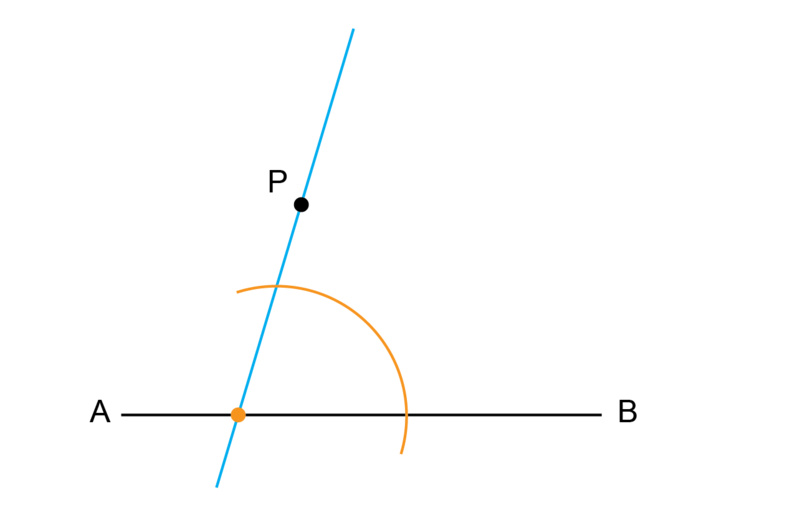3. Step 3: Draw the second arc from point $P$.

Keep the compass at the same width to draw the second arc. Put the compass point on point $P$ and draw the second arc above $P$. Make sure that the second arc goes lower than point $P$ on the right hand side.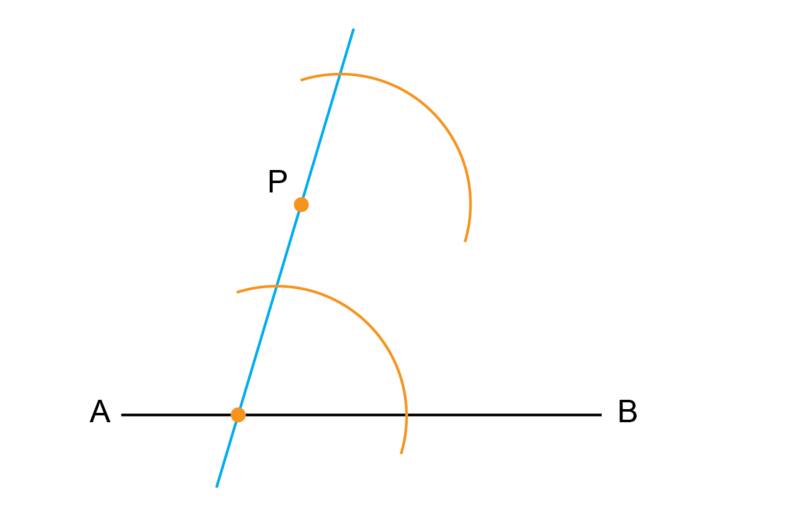4. Step 4: Use the compass to measure the distance between the two points where the first arc intersects the lines (the two purple dots).

Put the point of the compass where the first arc intersects the line $AB$ (at the purple point now labelled $R$). Put the pencil end of the compass where this arc intersects the line through $P$ (at the purple point now labelled $S$).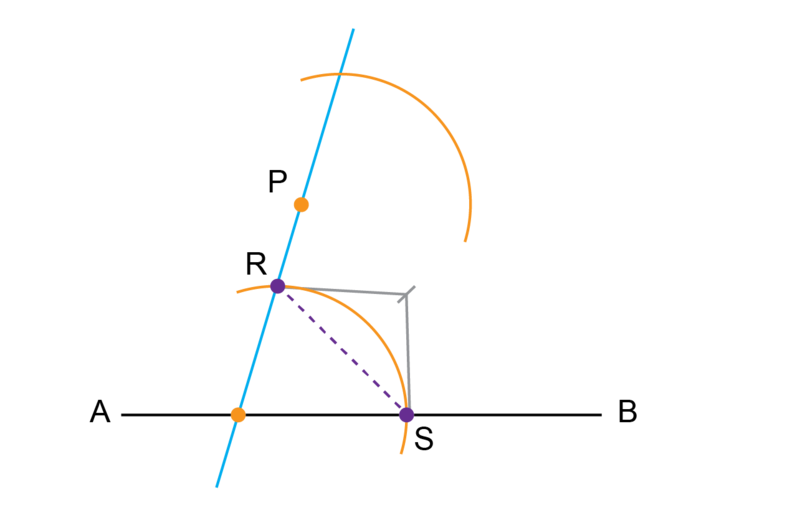IMPORTANT: Do not draw a line between point $R$ and point $S$. You are only measuring the distance.

5. Step 5: Mark the same length on the second arc.

Keep the compass in exactly the same position. Put the compass point on point $T$, and draw an arc to find point $Q$. Point $Q$ will be where this new arc intersects the second orange arc. The distance between point $T$ and point $Q$ should be exactly the same as the distance between point $R$ and point $S$.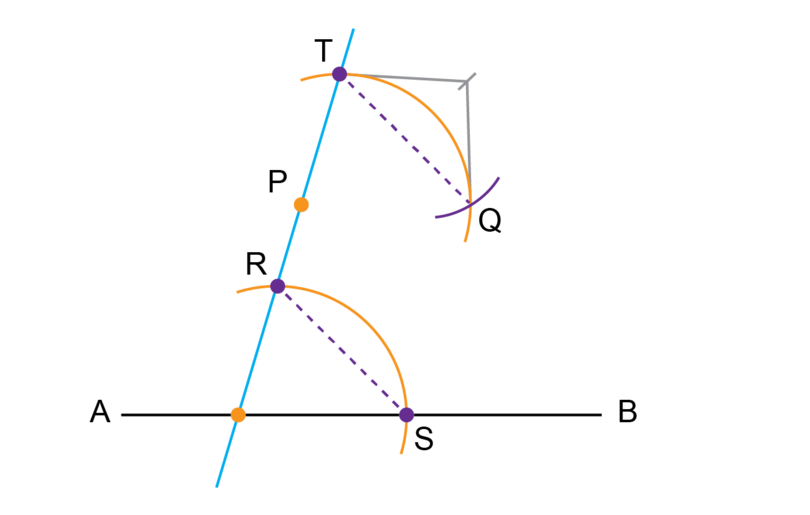IMPORTANT: Do not draw a line between point $T$ and point $Q$. You are only measuring off the correct distance.

6. Step 6: Draw a line through point $P$ and point $Q$.

When you draw a line through point $P$ and point $Q$, line $PQ$ will be parallel to line $AB$.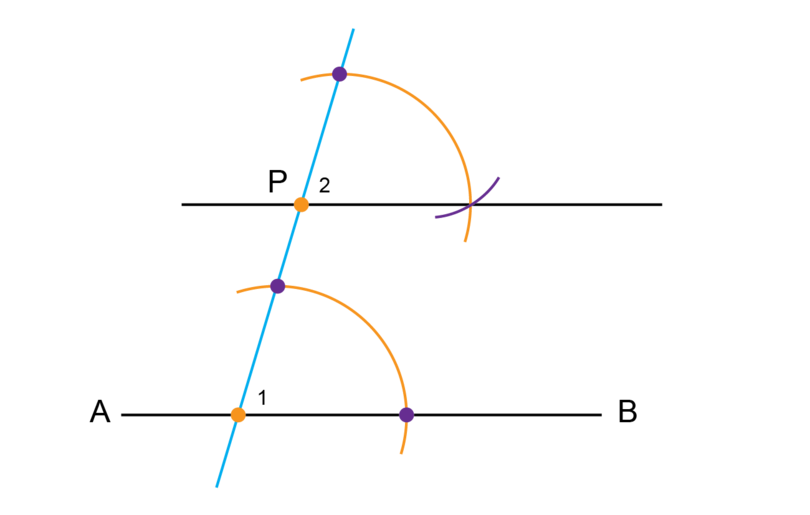7. Step 7: Check your construction.

Use a protractor to measure $\hat 1$ and $\hat 2$. If the two angles are equal to each other, your construction is accurate.

Remember, you can rotate your workbook to make it easier to work with a line that is not horizontal. Do not erase your construction lines after you have completed a construction, and always check your work by measuring the angles.

### Exercise 14.3: Construct a parallel line

1. Draw line $CD$ as shown below. Point $P$ is a point away from the line.Construct $PQ$ parallel to line $CD$.

Follow the Steps as shown in Worked example 14.3.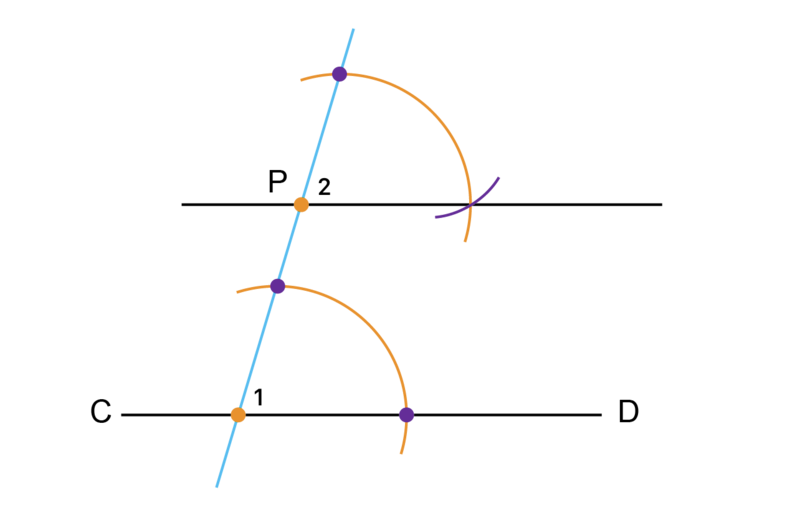If you have worked accurately, the two angles labelled $\hat{1}$ and $\hat{2}$ will be equal.

2. Draw line $EF$ as shown below. Point $P$ is a point away from the line.Construct $PQ$ parallel to line $EF$.

Follow the Steps as shown in Worked example 14.3.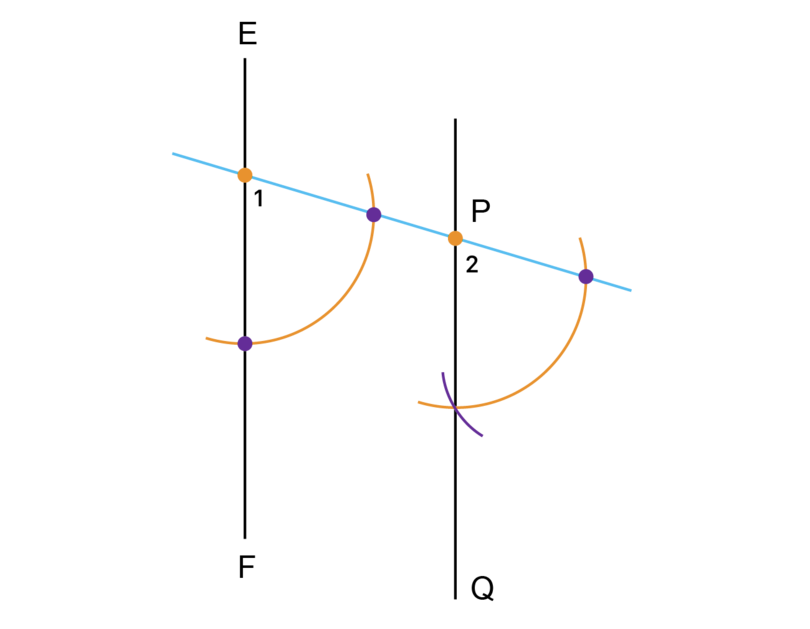If you have worked accurately, the two angles labelled $\hat{1}$ and $\hat{2}$ will be equal.

3. Draw line $MN$ as shown below. Point $P$ is a point away from the line.Construct $PQ$ parallel to line $MN$.

Follow the Steps as shown in Worked example 14.3.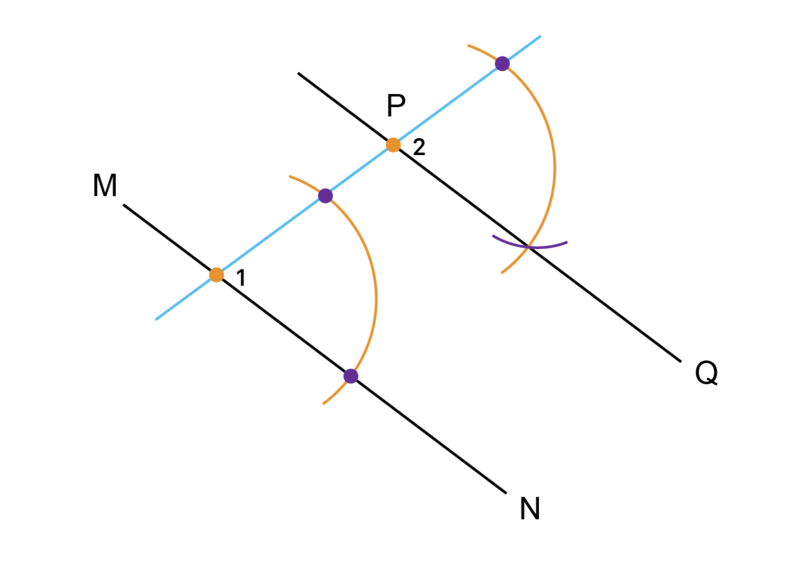If you have worked accurately, the two angles labelled $\hat{1}$ and $\hat{2}$ will be equal.

4. Draw line $ST$ as shown below. Point $P$ is a point away from the line.Construct $PQ$ parallel to line $ST$.

Follow the Steps as shown in Worked example 14.3.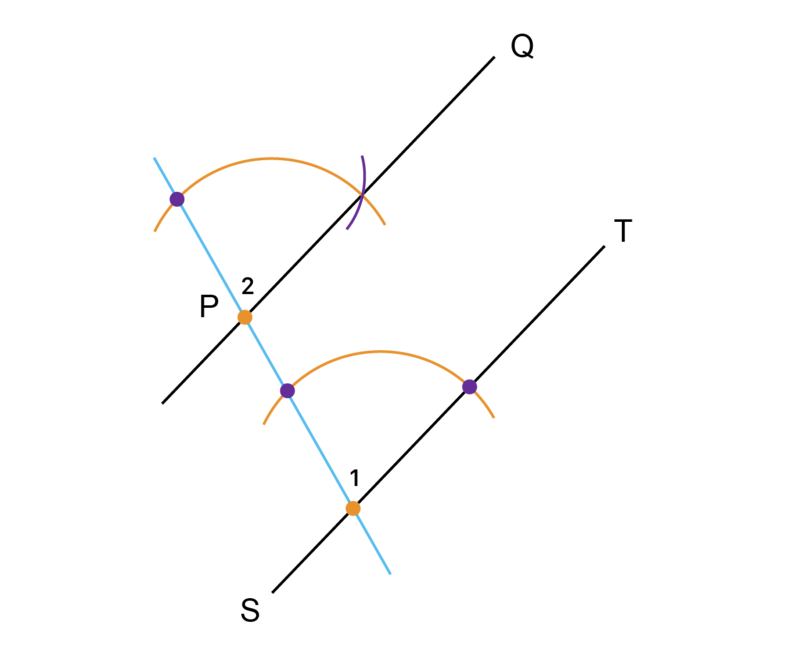If you have worked accurately the two angles labelled $\hat{1}$ and $\hat{2}$ will be equal.

## 14.3 Line bisector

A line segment is part of a straight line. A line segment has a beginning and an end. When we are asked to bisect a line segment, we are asked to divide the line segment into two equal parts.

The bisector is the line segment that divides the given line segment into two equal parts.

bisect When we bisect a line segment or an angle, we divide the line segment or the angle exactly in half.

bisector A bisector is the line segment that divides the given line segment into two equal parts.

### Worked example 14.4: Bisecting a line segment

You are given line segment $AB$ as shown in the diagram below.Bisect $AB$ and label the bisector $PQ$.

1. Step 1: Draw the first set of two arcs.

Open the compass so that the distance between the two points is more than half of the length of $AB$. Test this on line $AB$.

Put the compass on point $A$ (the blue dot) and draw two arcs as shown. One arc is above $AB$ and one arc is below $AB$.2. Step 2: Draw the second set of two arcs.

Keep the compass open at exactly the same width as for Step 1.

Put the compass on point $B$ (the orange dot) and draw two arcs as shown. One arc is above $AB$ and one arc is below $AB$.3. Step 3: Draw the bisector.

Label the points where the arcs of intersect as point $P$ and point $Q$.

Use a ruler and draw a line through point $P$ and point $Q$. $PQ$ is the bisector of line segment $AB$ at point $R$.4. Step 4: Check your work.

Measure the lengths of the two parts of line segment $AB$. If $AR$ is equal to $RB$, your construction is accurate.

Take note that if you measure the angles at $R$, you will find they are $90^{\circ}$. We say that the bisector is perpendicular to the original line segment. The symbol for perpendicular is $\perp$, so we write: $PQ \perp AB$.

When we do a construction to bisect a line, the bisector is always perpendicular to the line. This means we can call the bisector a "perpendicular line bisector ".

If you find it easier, you may draw two bigger arcs instead of four smaller arcs when you construct the bisector of a line segment, as shown in the diagram below.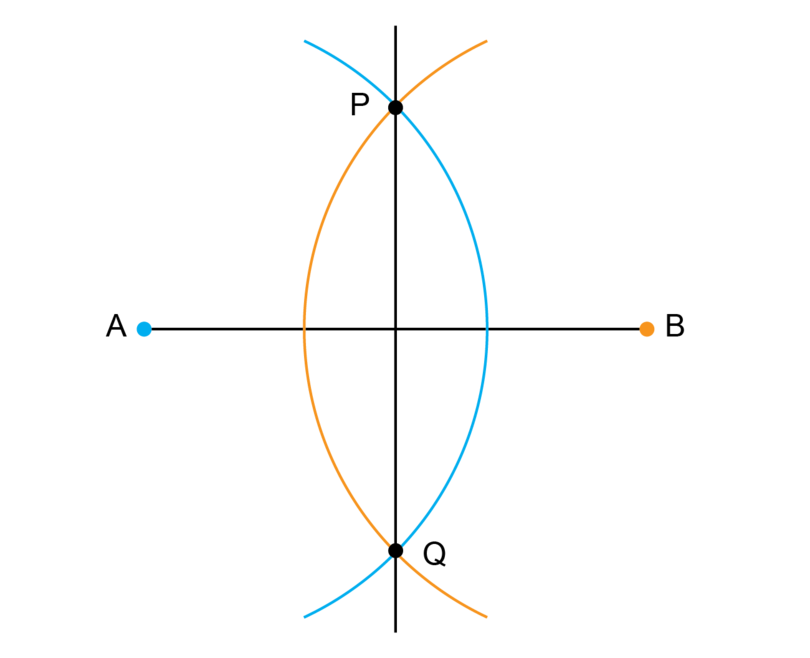### Exercise 14.4: Bisect a line segment

1. Draw line segment $CD$ as shown below.Bisect the line segment and label the bisector $PQ$.

Follow the Steps as shown in Worked example 14.4.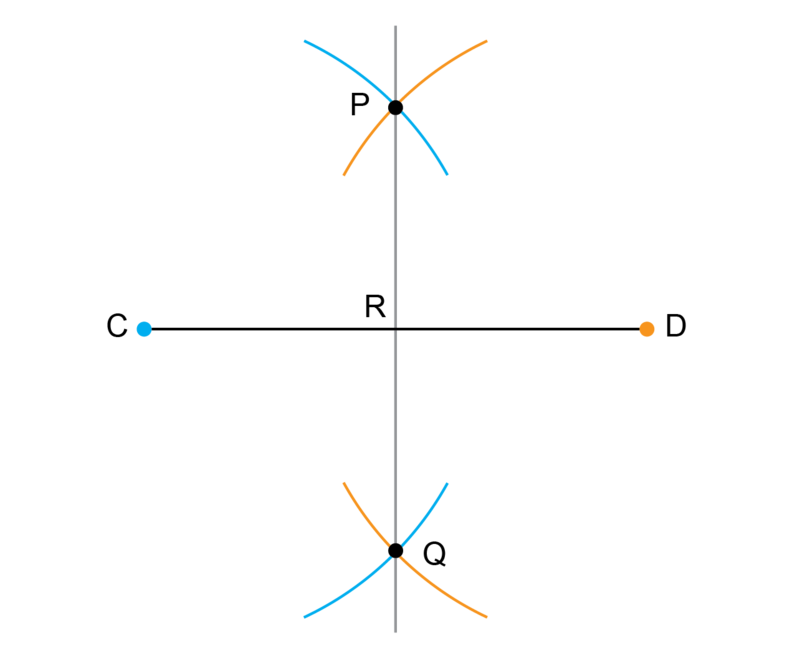If you have worked accurately, the length of line segment $CR$ will be the same as the length of line segment $RD$.

2. Draw line segment $EF$ as shown below.Bisect the line segment and label the bisector $PQ$.

Follow the Steps as shown in Worked example 14.4.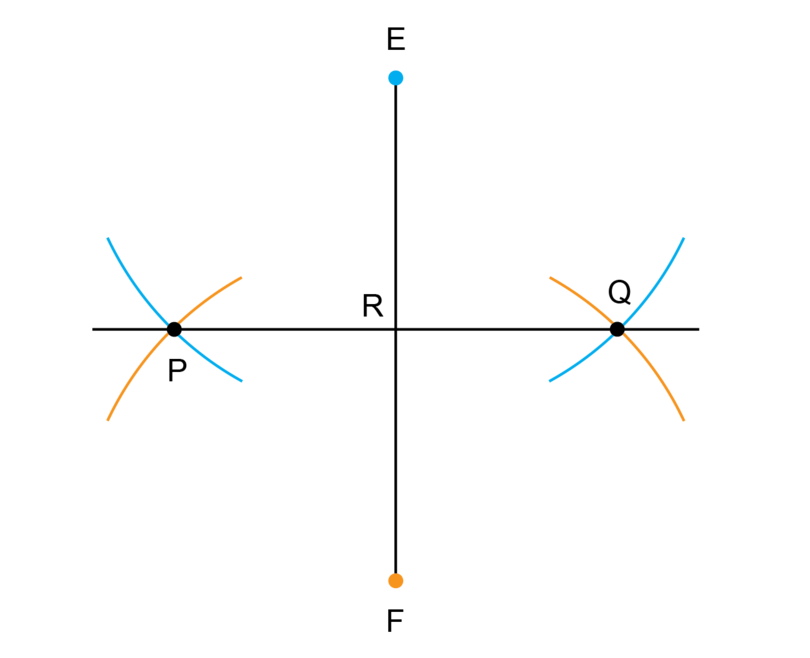If you have worked accurately, the length of line segment $ER$ will be the same as the length of line segment $RF$.

3. Draw line segment $MN$ as shown below.Bisect the line segment and label the bisector $PQ$. Remember to check your work.

Follow the Steps as shown in Worked example 14.4.If you have worked accurately, the length of line segment $MR$ will be the same as the length of line segment $RN$.

4. Draw line segment $ST$ as shown below.Bisect the line segment and label the bisector $PQ$.

Follow the Steps as shown in Worked example 14.4.If you have worked accurately, the length of line segment $SR$ will be the same as the length of line segment $RT$.

## 14.4 Sixty-degree angles

Here is a fun activity for you to do: Use a compass and draw a circle. Keep the position of the legs of the compass the same for the whole activity.

Make a dot anywhere on the circumference of the circle.Put the compass on the dot and start to make small arcs on the circumference of the circle:You will find that you can fit in exactly six of these small arcs:You know that a full turn (or revolution) is equal to $360^{\circ}$, so if we join the centre of the circle to each one of the six small arcs, we form six angles of $60^{\circ}$ each.If you are asked to construct an angle of $60^{\circ}$, you simply do a part of the fun activity that you have done above.

### Worked example 14.5: Constructing an angle of 60 degrees

You are given a line segment $BD$ as shown below. Construct $AB$ so that $C$ is a point on $AB$ and $A\hat{B}C$ = $60^{\circ}$.1. Step 1: Draw the first big arc.

Put the compass point at point $B$ (on the blue dot) and draw a big arc. Label point $C$ where the arc crosses line segment $BD$. Keep the compass at the same width for Step 2.2. Step 2: Draw a small arc that crosses the first big arc.

Keep the compass at the same width for Step 1. Put the compass point at point $C$ (on the orange dot) and make an arc to cross the big arc that you drew in Step 1.

Label the point of intersection $A$.3. Step 3: Complete the construction.

Use a ruler and draw a line segment from point $B$ through point $A$.4. Step 4: Check your work.

Use a protractor to measure $A\hat{B}C$. The angle should be equal to $60^{\circ}$.

When you construct an angle of $60^{\circ}$ you are actually constructing a triangle with three equal sides. In an equilateral triangle all three sides have equal lengths and each of the angles are equal to $60^{\circ}$.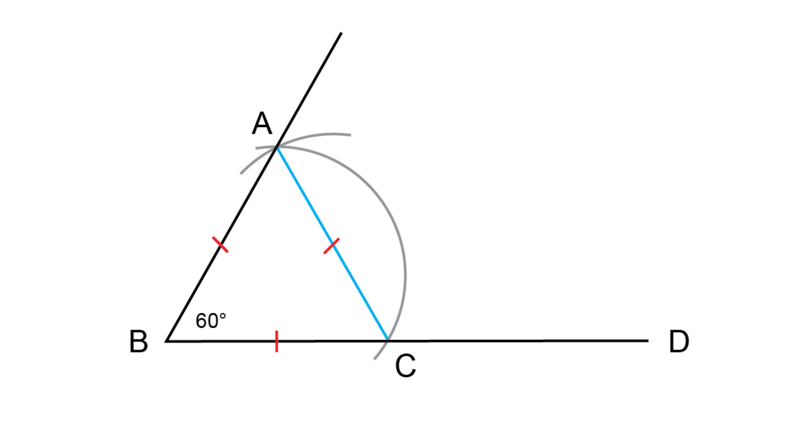### Exercise 14.5: Construct an angle of 60 degrees

1. Draw line segment $CD$ as shown below.Construct $FC$ so that $F\hat{C}D$ = $60^{\circ}$.

Follow the Steps as shown in Worked example 14.5.If you have worked accurately, $F\hat{C}D$ will be equal to $60^{\circ}$.

2. Draw line segment $EF$ as shown below.Construct $DE$ so that $D\hat{E}F$ = $60^{\circ}$.

Follow the Steps as shown in Worked example 14.5.If you have worked accurately, $D\hat{E}F$ will be equal to $60^{\circ}$.

3. Draw line segment $MN$ as shown below.Construct $LM$ so that $L\hat{M}N$ = $60^{\circ}$.

Follow the Steps as shown in Worked example 14.5.If you have worked accurately, $L\hat{M}N$ will be equal to $60^{\circ}$.

4. Draw line segment $ST$ as shown below.Construct $PS$ so that $P\hat{S}T$ = $60^{\circ}$.

Follow the Steps as shown in Worked example 14.5.If you have worked accurately, $P\hat{S}T$ will be equal to $60^{\circ}$.

## 14.5 Right angle

A right angle is an angle of 90 degrees. In real life we often see right angles. For example, the corners of your workbook are right angles, the corners of a door are right angles, and the corners of most tables are right angles.

When you are asked to construct an angle of $90^{\circ}$, you will again make use of the fun activity in which you made six small arcs on the circumference of a circle.

In this construction you will make only two arcs: you will mark where to form an angle of $60^{\circ}$ and where to form an angle of $120^{\circ}$.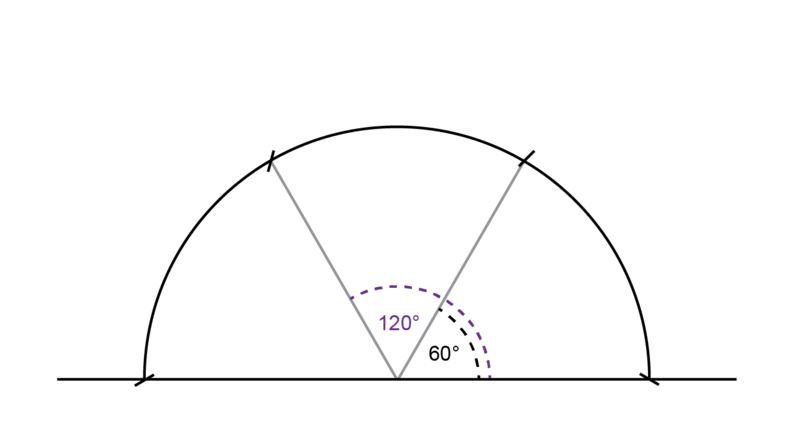An angle of $90^{\circ}$ is exactly halfway between $60^{\circ}$ and $120^{\circ}$, so you will construct the one arm of the right angle exactly in the middle of the arcs for $60^{\circ}$ and $120^{\circ}$.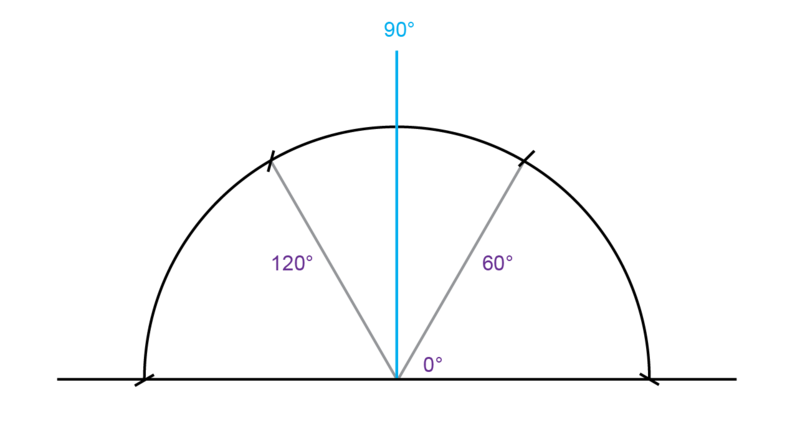### Worked example 14.6: Constructing a right angle

You are given a line segment $BD$ as shown below. Construct $AB$ so that $A\hat{B}C$ = $90^{\circ}$.1. Step 1: Draw the first big arc.

Put the compass point at point $B$ (on the blue dot) and draw a big arc. Label point $C$ where the arc intersects line segment $BD$.2. Step 2: Draw the first small arc for an angle of $60^{\circ}$.

Keep the compass at the same width for the following two arcs (Step 2 and Step 3).

Put the compass on point $C$ and make an arc on the big arc that was drawn in Step 1.

Label the point of intersection $P$. (Point $P$ shows the mark for an angle of $60^{\circ}$.)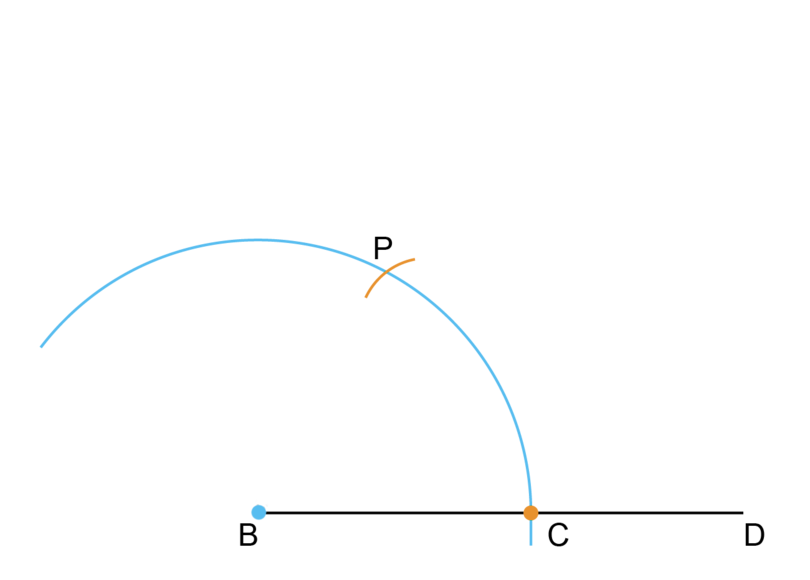3. Step 3: Draw the second small arc for an angle of $120^{\circ}$.

The compass should still be at exactly the same width as for the first big arc.

Put the compass on point $P$ and make another arc on the big arc that was drawn in Step 1.

Label the point of intersection point $Q$. (Point $Q$ shows the mark for an angle of $120^{\circ}$.)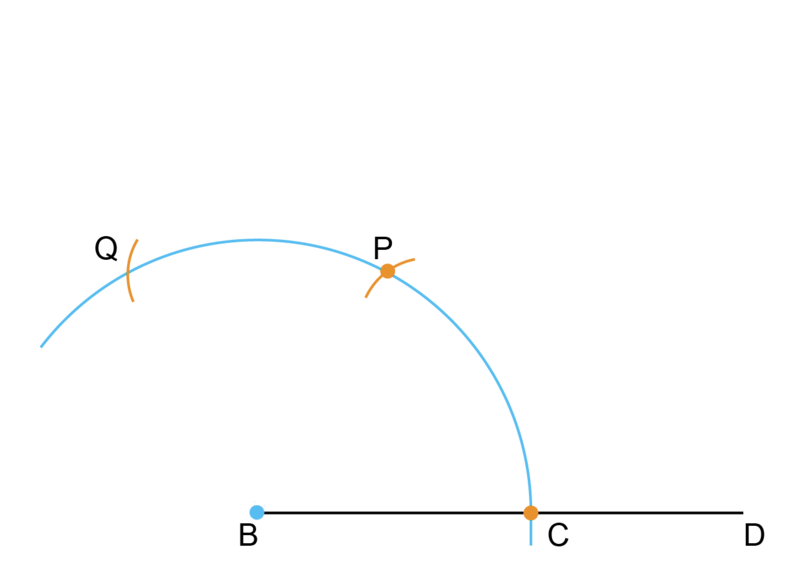4. Step 4: Draw the first arc to find the right angle.

We want to find the line for an angle of $90^{\circ}$ and we know that this line should be exactly in the middle between the arcs for the angles of $60^{\circ}$ and $120^{\circ}$.

The compass can now be less open, but it should stay at the same width for these next two arcs (Step 4 and Step 5).

Put the compass on point $P$ and make an arc as shown below.5. Step 5: Draw the second arc to find the right angle.

Keep the compass at the same width as for Step 4.

Put the compass on point $Q$ and draw an arc. Label the point of intersection between the two arcs $A$.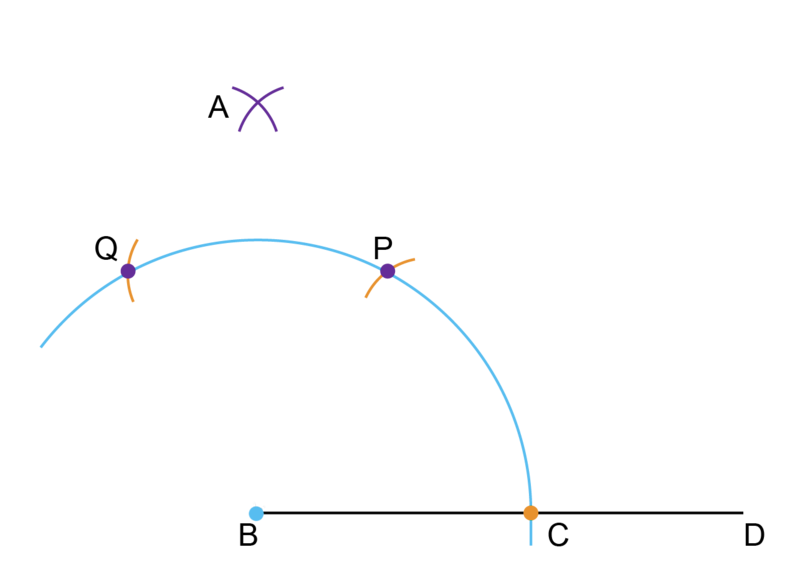6. Step 6: Complete the construction.

Use a ruler and draw a line segment from point $B$ and through point $A$.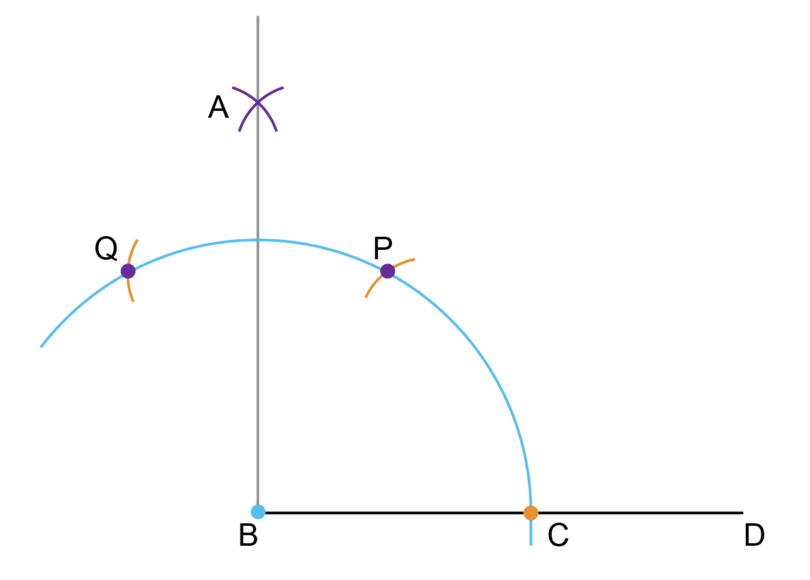7. Step 7: Check your work.

Use a protractor to measure $A\hat{B}C$. The angle should be equal to $90^{\circ}$.

### Exercise 14.6: Construct a right angle

1. Draw line segment $CD$ as shown below.Construct $FC$ so that $F\hat{C}D$ = $90^{\circ}$.

Follow the Steps as shown in Worked example 14.6.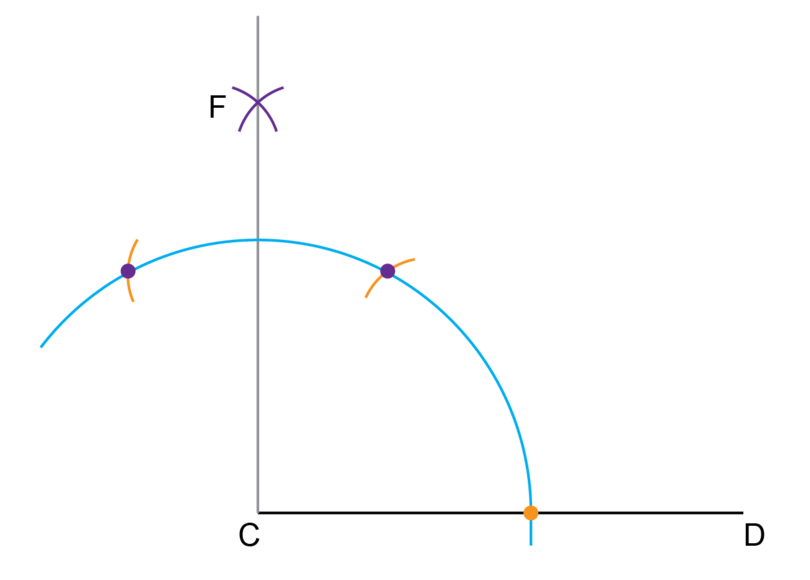If you have worked accurately, $F\hat{C}D$ will be equal to $90^{\circ}$.

2. Draw line segment $EF$ as shown below.Construct $DE$ so that $D\hat{E}F$ = $90^{\circ}$.

Follow the Steps as shown in Worked example 14.6.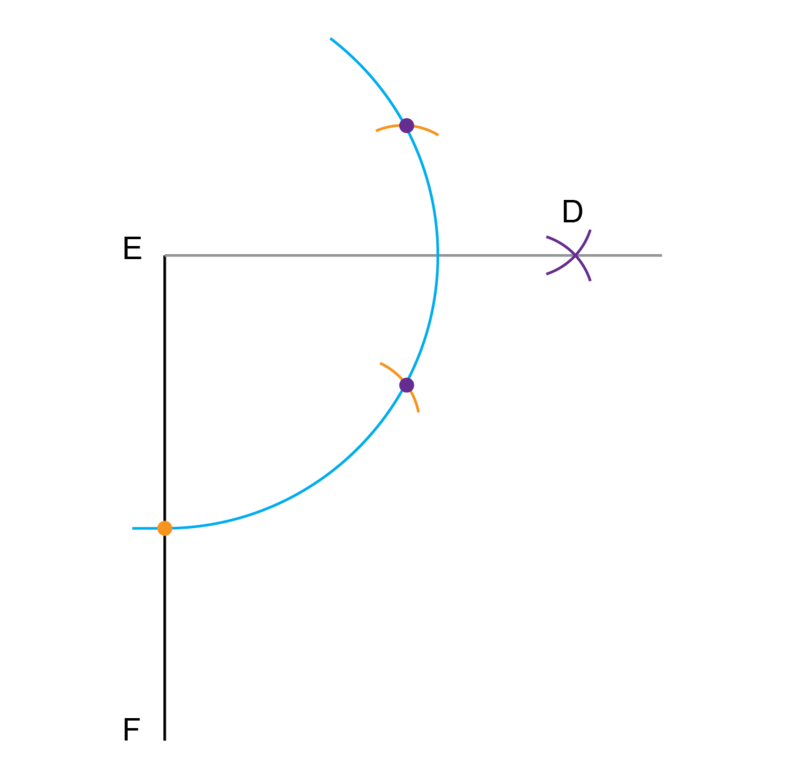If you have worked accurately, $D\hat{E}F$ will be equal to $90^{\circ}$.

3. Draw line segment $MN$ as shown below.Construct $LM$ so that $L\hat{M}N$ = $90^{\circ}$.

Follow the Steps as shown in Worked example 14.6.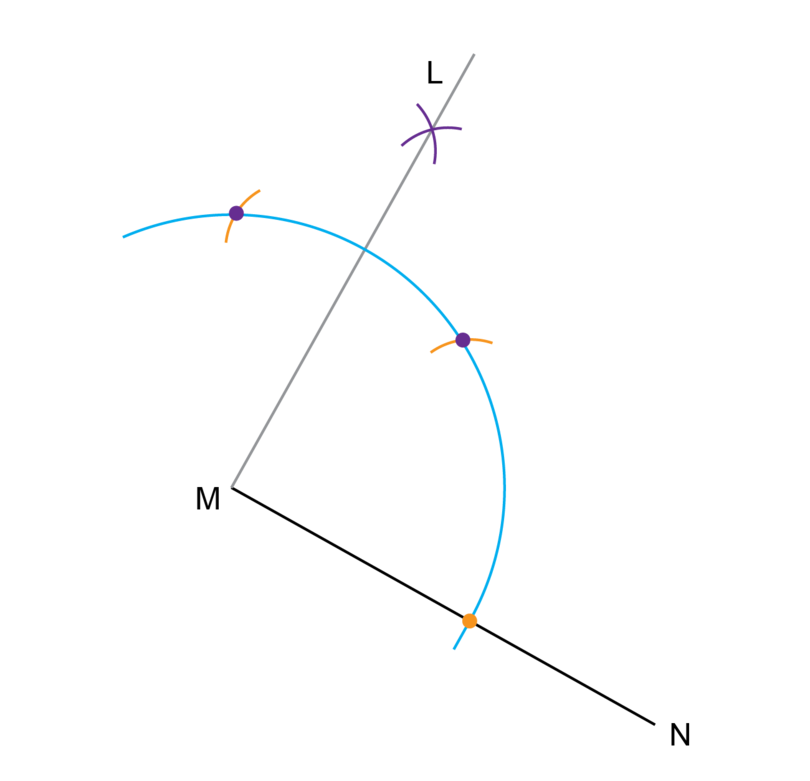If you have worked accurately, $L\hat{M}N$ will be equal to $90^{\circ}$.

4. Draw line segment $ST$ as shown below.Construct $PS$ so that $P\hat{S}T$ = $90^{\circ}$.

Follow the Steps as shown in Worked example 14.6.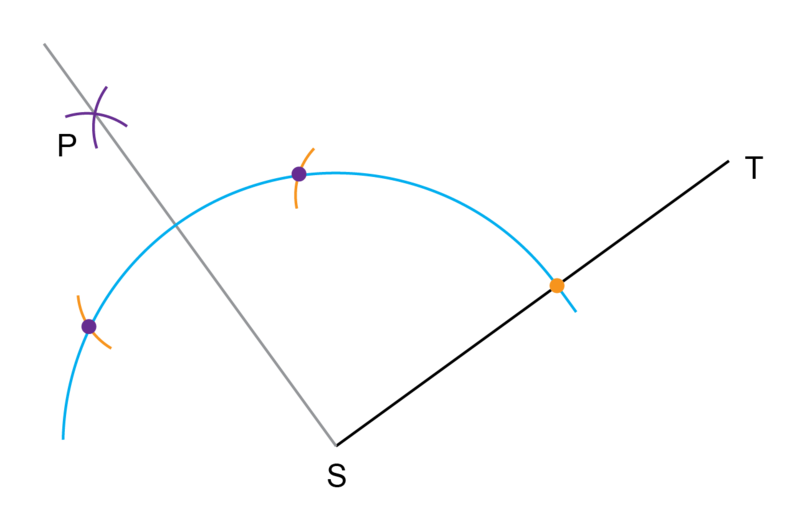If you have worked accurately, $P\hat{S}T$ will be equal to $90^{\circ}$.

## 14.6 Summary

• A construction in Mathematics is an accurate drawing of angles and lines.
• You are only allowed to use a straightedge and a pair of compasses with a pencil in it when you do a construction.
• You may use a ruler if you do not have a straightedge, but do not use the markings on the ruler.
• You will draw arcs in all the constructions. An arc is part of the circumference of a circle.
• You have to practise the following constructions:
• Construct a perpendicular line from a point on the line
• Construct a perpendicular line from a point away from the line
• Construct a parallel line through a given point
• Bisect a line segment
• Construct an angle of 60 degrees
• Construct a right angle (an angle of 90 degrees)
• Never erase the construction lines after you have completed a construction.